# 深度神经网络 (第六部分)。 神经网络分类器的融合: 引导聚合

8 八月 2018, 10:13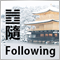0
3 099

### 1. 神经网络分类器的融合

Tin Kam Ho 在 2002 年出版的论文 "多个分类器合并: 课题与后续步骤" 中写道:

"现在我们寻找最佳的分类器集合，然后寻找最佳的合并方法，来替代寻找最佳的特征集合与最佳的分类器。 可以想象，我们很快就会寻找到最好的合并方法，然后是使用它们的最好方法。 如果我们不抓住机会回顾这一挑战所引发的根本问题，我们必然会陷入这种无休止的往复，拖着越来越复杂的合并方案和理论，逐渐忽视原有的问题。"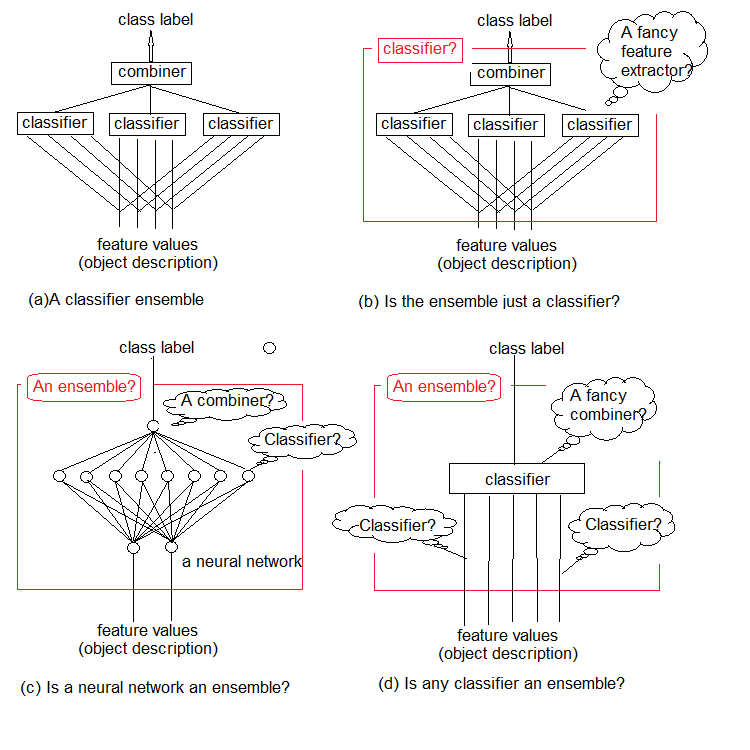• 减少差异 — 引导聚合 (bagging);
• 减少偏见 — 提振 (boosting);
• 改善预测 — 堆叠 (stacking)

• 构造融合的并行方法，其中基本模型是并行生成的 (例如，随机森林)。 我们的思路是 利用基础模型之间的独立性 并通过平均值来减少误差。 因此，对于模型的主要要求 — 互关联性低，多元化高。
• 顺序融合方法，其基本模型是顺序生成的 (例如，AdaBoost，XGBoost)。 这里的主要思想是 利用基础模型之间的依赖性。 在此，为先前分类有误的示例分配较高权重，可以提高整体品质。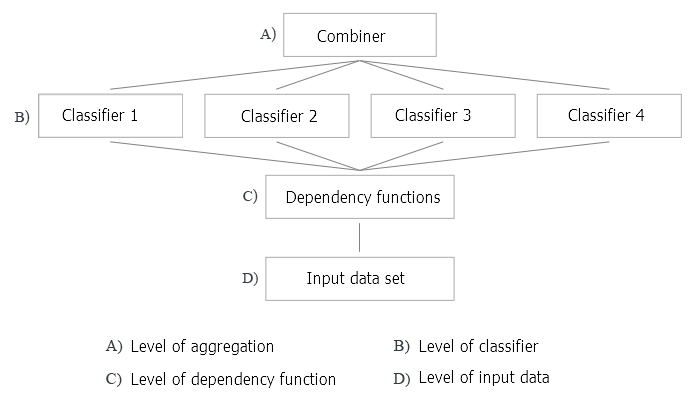#### 1. 合并器

• 非可训练。 这种方法的一个例子是简单的 "多数表决"。
• 可训练。 该组包括 "加权多数表决" 和 "朴素贝叶斯" ，以及 "分类器选择" 方法，其中给定对象的决策是由融合的一个分类器做出的。
• 元分类器。 基础分类器的输出被认作训练新分类器的输入，其会变为合并器。 这种方法被称为 "复杂泛化" ，"通过训练泛化"，或简称为 "堆叠"。 为元分类器构建训练集合是该合并器的主要问题之一。

#### 3. 多元化

• 操纵训练参数。 在训练单体基础分类器时使用不同的方法和参数。 例如，可以用不同的随机变量为每个基础分类器的神经网络隐藏层中的神经元初始化权重。 也可以随机设置超参数。
• 操纵样本 — 从训练集合中为融合的每个成员取一个自定义引导样本。
• 操纵预测器 — 为每个基础分类器准备一组随机确定的预测器。 这就是所谓的训练集合垂直切分。

#### 4. 融合大小

• 该数量是提前预留的
• 这个数字是在训练过程中设定的
• 分类器过量生产然后被选中

#### 5. 多功能性 (相对于基础分类器)

• 仅能使用一个基础分类器的特定模型;
• 可以使用任意基础分类器模型。

• 决策优化是指为基础分类器的固定融合选择合并器 (图例 2 中的级别 A)。
• 备用的覆盖优化是指利用固定合并器创建的多元化基础分类器 (图例 2 中的级别 B，C 和 D)。

### 2. 引导聚合 (Bagging)

1. 从训练集合中提取引导样本;
2. 每个分类器都在自己的样本上进行训练;
3. 来自单体分类器的各个输出被合并成一个类标签。 如果单体输出拥有类标签的形式，则使用简单多数表决。 如果分类器的输出是连续变量，则应用平均值，或者将变量转换为类标签，然后进行简单多数表决。

#### B: 分类器级别

• 我们使用不同或相同的分类器吗？ 在融合方法中使用相同的分类器。
• 哪个分类器作为基础分类器？ 我们使用 ELM (极限学习机)。

• 需要多少个分类器？ 我们取 500，然后修剪融合。
• 是否使用分类器的并行或顺序训练？ 我们使用并行训练，同时针对所有分类器进行。
• 基础分类器的哪些参数可以操纵？ 隐藏层数量，激活函数，训练集合的样本大小。 所有这些参数都需要优化。

#### C: 辨别规则的功能级别

• 使用所有预测器还是仅使用每个分类器的单独子集？ 所有分类器都使用一个预测器子集。 但是可以优化预测器的数量。
• 如何选择这样一个子集？ 在这种情况下，使用特殊算法。

#### D: 输入数据及其操纵的级别

1. 生成源数据集合
2. 按照信息重要性安排预测器
3. 训练和测试融合分类器
4. 合并单体分类器的输出 (平均/表决)
5. 融合修剪及其方法
6. 搜索融合分类品质的度量衡
7. 确定融合成员的优化参数。 方法
8. 使用优化参数训练和测试融合

#### 生成源数据集合

```#----准备-------------
library(anytime)
library(rowr)
library(elmNN)
library(rBayesianOptimization)
library(foreach)
library(magrittr)
library(clusterSim)
#source(file = "FunPrepareData.R")
#source(file = "FUN_Ensemble.R")
#---准备----
evalq({
dt <- PrepareData(Data, Open, High, Low, Close, Volume)
DT <- SplitData(dt, 4000, 1000, 500, 250, start = 1)
pre.outl <- PreOutlier(DT\$pretrain)
DTcap <- CappingData(DT, impute = T, fill = T, dither = F, pre.outl = pre.outl)
preproc <- PreNorm(DTcap, meth = meth)
DTcap.n <- NormData(DTcap, preproc = preproc)
}, env)
```

```#---数据 X-------------
evalq({
list(
pretrain = list(
x = DTcap.n\$pretrain %>% dplyr::select(-c(Data, Class)) %>% as.data.frame(),
y = DTcap.n\$pretrain\$Class %>% as.numeric() %>% subtract(1)
),
train = list(
x = DTcap.n\$train %>% dplyr::select(-c(Data, Class)) %>% as.data.frame(),
y = DTcap.n\$train\$Class %>% as.numeric() %>% subtract(1)
),
test = list(
x = DTcap.n\$val %>% dplyr::select(-c(Data, Class)) %>% as.data.frame(),
y = DTcap.n\$val\$Class %>% as.numeric() %>% subtract(1)
),
test1 = list(
x = DTcap.n\$test %>% dplyr::select(-c(Data, Class)) %>% as.data.frame(),
y = DTcap.n\$test\$Class %>% as.numeric() %>% subtract(1)
)
) -> X
}, env)
```

#### 按照信息重要性安排预测器

```require(clusterSim)
evalq({
numFeature <- 10
HINoV.Mod(x = X\$pretrain\$x %>% as.matrix(), type = "metric", s = 1, 4,
distance =  NULL, # "d1" - Manhattan, "d2" - Euclidean,
#"d3" - Chebychev (max), "d4" - squared Euclidean,
#"d5" - GDM1, "d6" - Canberra, "d7" - Bray-Curtis
method = "kmeans" ,#"kmeans" (default) , "single",
#"ward.D", "ward.D2", "complete", "average", "mcquitty",
#"median", "centroid", "pam"
Index = "cRAND") -> r
r\$stopri[ ,1] %>% head(numFeature) -> bestF
}, env)
print(env\$r\$stopri)
[,1]      [,2]
[1,]    5 0.9242887
[2,]   11 0.8775318
[3,]    9 0.8265240
[4,]    3 0.6093157
[5,]    6 0.6004115
[6,]   10 0.5730556
[7,]    1 0.5722479
[8,]    7 0.4730875
[9,]    4 0.3780357
[10,]    8 0.3181561
[11,]    2 0.2960231
[12,]   12 0.1009184
```

```> colnames(env\$X\$pretrain\$x)[env\$bestF]
 "v.fatl" "v.rbci" "v.ftlm" "rbci"   "v.satl" "v.stlm" "ftlm"
 "v.rftl" "pcci"   "v.rstl"
```

#### 创建，训练和测试融合

1. - sig: sigmoid
2. - sin: sine
4. - hardlim: hard-limit
5. - hardlims: symmetric hard-limit
6. - satlins: satlins
7. - tansig: tan-sigmoid
8. - tribas: triangular basis
9. - poslin: positive linear
10. - purelin: linear

```#---3-----Train----------------------------
evalq({
n <- 500
r <- 7
nh <- 5
Xtrain <- X\$pretrain\$x[ , bestF]
Ytrain <- X\$pretrain\$y
Ens <- foreach(i = 1:n, .packages = "elmNN") %do% {
idx <- rminer::holdout(Ytrain, ratio = r/10, mode = "random")\$tr
elmtrain(x = Xtrain[idx, ], y = Ytrain[idx],
nhid = nh, actfun = "sin")
}
}, env)
```

```#---4-----predict-------------------
evalq({
Xtest <- X\$train\$x[ , bestF]
Ytest <- X\$train\$y
foreach(i = 1:n, .packages = "elmNN", .combine = "cbind") %do% {
predict(Ens[[i]], newdata = Xtest)
} -> y.pr #[ ,n]
}, env)
```

#### 合并分类器的单体输出。 方法 (平均/表决)

• 类标签
• 按 classes >2 分类时，类标签排序
• 连续数值预测/支持度。

#### 融合修剪。 方法

• 基于顺序的修剪 — 按特定品质得分排行中选择融合:
• 降低误差修剪 — 按分类误差对分类器进行排序，并选择若干最好的 (误差最小);
• kappa 修剪 — 根据 Kappa 统计信息对融合成员进行排序，选择得分最低的所需数字。
• 基于集群的修剪 — 通过任意方法对融合的预测结果进行集群，之后自每个集群里选择若干代表。 集群方法:
• 分区 (partitioning) (例如 SOM, k-mean);
• 分层 (hierarchical);
• 基于密度 (density-based) (例如, dbscan);
• 基于 GMM。
• 基于优化的修剪 — 运用进化或遗传算法选择最佳。

• 合并方法 — 平均和简单多数表决;
• 度量衡 — 准确率 (Accuracy), 精确率 (Precision), 召回率 (Recall) 和 F1;
• 修剪 — 从基于 mean(F1) 的分类误差排序的融合中进行选择。

a) 确定融合的最佳单体分类器

```#---5-----best----------------------
evalq({
numEns <- 3
foreach(i = 1:n, .combine = "c") %do% {
ifelse(y.pr[ ,i] > 0.5, 1, 0) -> Ypred
Evaluate(actual = Ytest, predicted = Ypred)\$Metrics\$F1 %>%
mean()
} -> Score
Score %>% order(decreasing = TRUE) %>% head((numEns*2 + 1)) -> bestNN
Score[bestNN] %>% round(3)
}, env)
 0.720 0.718 0.718 0.715 0.713 0.713 0.712
```

b) 平均

```#---6----test averaging(test)--------
evalq({
n <- len(Ens)
Xtest <- X\$test\$x[ , bestF]
Ytest <- X\$test\$y
foreach(i = 1:n, .packages = "elmNN", .combine = "+") %:%
when(i %in% bestNN) %do% {
predict(Ens[[i]], newdata = Xtest)} %>%
divide_by(length(bestNN)) -> ensPred
ifelse(ensPred > 0.5, 1, 0) -> ensPred
Evaluate(actual = Ytest, predicted = ensPred)\$Metrics[ ,2:5] %>%
round(3)
}, env)
Accuracy Precision Recall    F1
0     0.75     0.723  0.739 0.731
1     0.75     0.774  0.760 0.767
```

```#--6.1 ---test averaging(test1)---------
evalq({
n <- len(Ens)
Xtest <- X\$test1\$x[ , bestF]
Ytest <- X\$test1\$y
foreach(i = 1:n, .packages = "elmNN", .combine = "+") %:%
when(i %in% bestNN) %do% {
predict(Ens[[i]], newdata = Xtest)} %>%
divide_by(length(bestNN)) -> ensPred
ifelse(ensPred > 0.5, 1, 0) -> ensPred
Evaluate(actual = Ytest, predicted = ensPred)\$Metrics[ ,2:5] %>%
round(3)
}, env)
Accuracy Precision Recall    F1
0    0.745     0.716  0.735 0.725
1    0.745     0.770  0.753 0.761
```

c) 简单多数表决

```#--7 --test--voting(test)--------------------
evalq({
n <- len(Ens)
Xtest <- X\$test\$x[ , bestF]
Ytest <- X\$test\$y
foreach(i = 1:n, .packages = "elmNN", .combine = "cbind") %:%
when(i %in% bestNN) %do% {
predict(Ens[[i]], newdata = Xtest)
} %>%
apply(2, function(x) ifelse(x > 0.5, 1, -1)) %>%
apply(1, function(x) sum(x)) -> vot
ifelse(vot > 0, 1, 0) -> ClVot
Evaluate(actual = Ytest, predicted = ClVot)\$Metrics[ ,2:5] %>%
round(3)
}, env)
Accuracy Precision Recall    F1
0    0.745     0.716  0.735 0.725
1    0.745     0.770  0.753 0.761
```

```#--7.1 --test--voting(test1)--------------------
evalq({
n <- len(Ens)
Xtest <- X\$test1\$x[ , bestF]
Ytest <- X\$test1\$y
foreach(i = 1:n, .packages = "elmNN", .combine = "cbind") %:%
when(i %in% bestNN) %do% {
predict(Ens[[i]], newdata = Xtest)
} %>%
apply(2, function(x) ifelse(x > 0.5, 1, -1)) %>%
apply(1, function(x) sum(x)) -> vot
ifelse(vot > 0, 1, 0) -> ClVot
Evaluate(actual = Ytest, predicted = ClVot)\$Metrics[ ,2:5] %>%
round(3)
}, env)
Accuracy Precision Recall    F1
0    0.761     0.787  0.775 0.781
1    0.761     0.730  0.743 0.737
```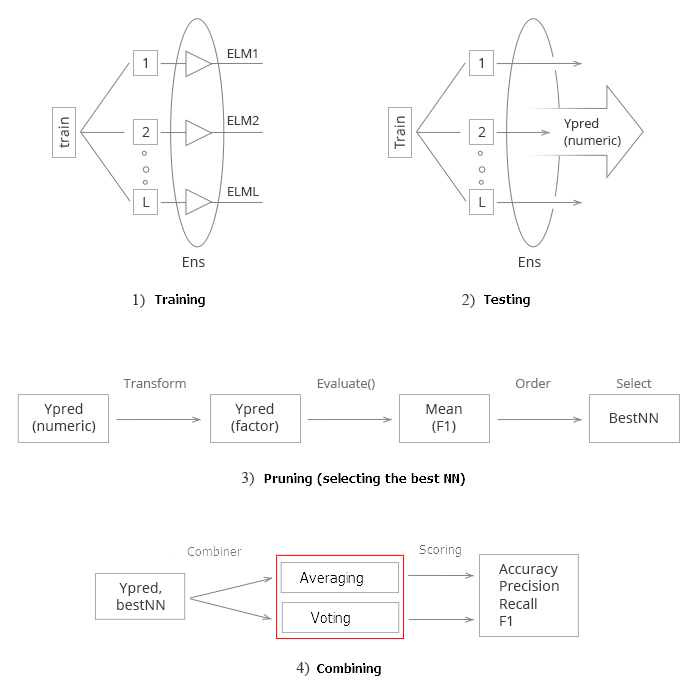1. 训练融合。 从训练集合中基于随机样本 (bootstrap) 训练 L 神经网络。 获得经过训练的神经网络融合。

2. 在测试集合上测试神经网络的融合。 获得单体分类器的连续预测。

3. 修剪融合，依据某个分类品质标准选择最佳的 n。 在此例中, 它就是 mean(F1).

4. 使用最佳单体分类器的连续预测，在平均或简单多数表决的帮助下将它们合并起来。 之后，确定度量衡。

• 优化融合 (贝叶斯优化器) 的单体分类器的超参数。
• DNN 将用作融合单体输出的合并器。 通过学习进行泛化。

#### 确定融合单体分类器的优化参数。 方法

ELM 的这个特性非常适合创建融合。 在融合中，我们不仅用随机值初始化每个分类器的权重，而且还为每个分类器提供单独的随机生成的训练样本。

```#----准备-------------
library(anytime)
library(rowr)
library(elmNN)
library(rBayesianOptimization)
library(foreach)
library(magrittr)
library(clusterSim)
library(doRNG)
#source(file = "FunPrepareData.R")
#source(file = "FUN_Ensemble.R")
#---准备----
evalq({
dt <- PrepareData(Data, Open, High, Low, Close, Volume)
DT <- SplitData(dt, 4000, 1000, 500, 250, start = 1)
pre.outl <- PreOutlier(DT\$pretrain)
DTcap <- CappingData(DT, impute = T, fill = T, dither = F, pre.outl = pre.outl)
preproc <- PreNorm(DTcap, meth = meth)
DTcap.n <- NormData(DTcap, preproc = preproc)
#--1-Data X-------------
list(
pretrain = list(
x = DTcap.n\$pretrain %>% dplyr::select(-c(Data, Class)) %>% as.data.frame(),
y = DTcap.n\$pretrain\$Class %>% as.numeric() %>% subtract(1)
),
train = list(
x = DTcap.n\$train %>% dplyr::select(-c(Data, Class)) %>% as.data.frame(),
y = DTcap.n\$train\$Class %>% as.numeric() %>% subtract(1)
),
test = list(
x = DTcap.n\$val %>% dplyr::select(-c(Data, Class)) %>% as.data.frame(),
y = DTcap.n\$val\$Class %>% as.numeric() %>% subtract(1)
),
test1 = list(
x = DTcap.n\$test %>% dplyr::select(-c(Data, Class)) %>% as.data.frame(),
y = DTcap.n\$test\$Class %>% as.numeric() %>% subtract(1)
)
) -> X
#---2--bestF-----------------------------------
#require(clusterSim)
numFeature <- 10
HINoV.Mod(x = X\$pretrain\$x %>% as.matrix(), type = "metric", s = 1, 4,
distance =  NULL, # "d1" - Manhattan, "d2" - Euclidean,
#"d3" - Chebychev (max), "d4" - squared Euclidean,
#"d5" - GDM1, "d6" - Canberra, "d7" - Bray-Curtis
method = "kmeans" ,#"kmeans" (default) , "single",
#"ward.D", "ward.D2", "complete", "average", "mcquitty",
#"median", "centroid", "pam"
Index = "cRAND") %\$% stopri[ ,1] -> orderX
}, env)
```

```#---3-----Train----------------------------
evalq({
Xtrain <- X\$pretrain\$x[ , bestF]
Ytrain <- X\$pretrain\$y
n <- 500
r <- 7
nh <- 5
k <- 1
rng <- RNGseq(n, 12345)
Ens <- foreach(i = 1:n, .packages = "elmNN") %do% {
rngtools::setRNG(rng[[k]])
k <- k + 1
idx <- rminer::holdout(Ytrain, ratio = r/10, mode = "random")\$tr
elmtrain(x = Xtrain[idx, ], y = Ytrain[idx],
nhid = nh, actfun = "sin")
}
}, env)
```

```#---4-----predict-------------------
evalq({
Xtest <- X\$train\$x[ , bestF]
Ytest <- X\$train\$y
foreach(i = 1:n, .packages = "elmNN", .combine = "cbind") %do% {
predict(Ens[[i]], newdata = Xtest)
} -> y.pr #[ ,n]
}, env)
#---5-----best----------------------
evalq({
numEns <- 3
foreach(i = 1:n, .combine = "c") %do% {
ifelse(y.pr[ ,i] > 0.5, 1, 0) -> Ypred
Evaluate(actual = Ytest, predicted = Ypred)\$Metrics\$F1 %>%
mean()
} -> Score
Score %>% order(decreasing = TRUE) %>% head((numEns*2 + 1)) -> bestNN
Score[bestNN] %>% round(3)
}, env)
#  0.723 0.722 0.722 0.719 0.716 0.714 0.713
#---6----test averaging(test)--------
evalq({
n <- len(Ens)
Xtest <- X\$test\$x[ , bestF]
Ytest <- X\$test\$y
foreach(i = 1:n, .packages = "elmNN", .combine = "+") %:%
when(i %in% bestNN) %do% {
predict(Ens[[i]], newdata = Xtest)} %>%
divide_by(length(bestNN)) -> ensPred
ifelse(ensPred > 0.5, 1, 0) -> ensPred
Evaluate(actual = Ytest, predicted = ensPred)\$Metrics[ ,2:5] %>%
round(3)
}, env)
# Accuracy Precision Recall F1
# 0 0.75 0.711 0.770 0.739
# 1 0.75 0.790 0.734 0.761
#--7 --test--voting(test)--------------------
evalq({
n <- len(Ens)
Xtest <- X\$test\$x[ , bestF]
Ytest <- X\$test\$y
foreach(i = 1:n, .packages = "elmNN", .combine = "cbind") %:%
when(i %in% bestNN) %do% {
predict(Ens[[i]], newdata = Xtest)
} %>%
apply(2, function(x) ifelse(x > 0.5, 1, -1)) %>%
apply(1, function(x) sum(x)) -> vot
ifelse(vot > 0, 1, 0) -> ClVot
Evaluate(actual = Ytest, predicted = ClVot)\$Metrics[ ,2:5] %>%
round(3)
}, env)
# Accuracy Precision Recall F1
# 0 0.749 0.711 0.761 0.735
# 1 0.749 0.784 0.738 0.760
```

• 输入数据中预测器的数量;
• 用于训练的样本的大小;
• 隐藏层中的神经元数量;
• 激活函数。

```evalq({
#type of activation function.
Fact <- c("sig", #: sigmoid
"sin", #: sine
"hardlim", #: hard-limit
"hardlims", #: symmetric hard-limit
"satlins", #: satlins
"tansig", #: tan-sigmoid
"tribas", #: triangular basis
"poslin", #: positive linear
"purelin") #: linear
bonds <- list(
numFeature = c(3L, 12L),
r = c(1L, 10L),
nh <- c(1L, 50L),
fact = c(1L, 10L)
)
}, env)
```

• numFeature — 作为输入的预测器的数量; 最小 3，最大 12;
• r — 引导中使用的训练集合的比例。 在计算之前，将其除以 10。
• nh — 隐藏层中的神经元数量; 最小 1，最大 50。
• fact — Fact 向量中的激活函数的索引。

```#---Fitnes -FUN-----------
evalq({
Ytrain <- X\$pretrain\$y
Ytest <- X\$train\$y
Ytest1 <- X\$test\$y
n <- 500
numEns <- 3
fitnes <- function(numFeature, r, nh, fact){
Xtrain <- X\$pretrain\$x[ , bestF]
k <- 1
rng <- RNGseq(n, 12345)
#---train---
Ens <- foreach(i = 1:n, .packages = "elmNN") %do% {
rngtools::setRNG(rng[[k]])
idx <- rminer::holdout(Ytrain, ratio = r/10, mode = "random")\$tr
k <- k + 1
elmtrain(x = Xtrain[idx, ], y = Ytrain[idx],
nhid = nh, actfun = Fact[fact])
}
#---predict---
Xtest <- X\$train\$x[ , bestF]
foreach(i = 1:n, .packages = "elmNN", .combine = "cbind") %do% {
predict(Ens[[i]], newdata = Xtest)
} -> y.pr #[ ,n]
#---best---
foreach(i = 1:n, .combine = "c") %do% {
ifelse(y.pr[ ,i] > 0.5, 1, 0) -> Ypred
Evaluate(actual = Ytest, predicted = Ypred)\$Metrics\$F1 %>%
mean()
} -> Score
Score %>% order(decreasing = TRUE) %>%
#---test-aver--------
Xtest1 <- X\$test\$x[ , bestF]
foreach(i = 1:n, .packages = "elmNN", .combine = "+") %:%
when(i %in% bestNN) %do% {
predict(Ens[[i]], newdata = Xtest1)} %>%
divide_by(length(bestNN)) -> ensPred
ifelse(ensPred > 0.5, 1, 0) -> ensPred
Evaluate(actual = Ytest1, predicted = ensPred)\$Metrics\$F1 %>%
mean() %>% round(3) -> Score
return(list(Score = Score, Pred = ensPred))
}
}, env)
```

n — 融合中神经网络的数量;

numEns — 最佳单体分类器的数量 (numEns*2 + 1)，其预测将被合并。

```#----------
evalq(
system.time(
res <- fitnes(numFeature = 10, r = 7, nh = 5, fact = 2)
)
, env)
user  system elapsed
8.65    0.19    7.86
```

```> env\$res\$Score
 0.761
```

```#------
evalq(
OPT_Res <- BayesianOptimization(fitnes, bounds = bonds,
init_grid_dt = NULL, init_points = 10,
n_iter = 20, acq = "ucb", kappa = 2.576,
eps = 0.0, verbose = TRUE)
, envir = env)
Best Parameters Found:
Round = 23  numFeature = 8.0000  r = 3.0000 nh = 3.0000  fact = 7.0000        Value = 0.7770
```

```evalq({
OPT_Res %\$% History %>% dplyr::arrange(desc(Value)) %>% head(10) %>%
dplyr::select(-Round) -> best.init
best.init
}, env)
numFeature r nh fact Value
1           8 3  3    7 0.777
2           8 1  5    7 0.767
3           8 3  2    7 0.760
4          10 7  9    8 0.759
5           8 5  4    7 0.758
6           8 2  7    8 0.756
7           8 6  9    7 0.755
8           8 3  4    8 0.754
9           9 2 13    9 0.752
10         11 2 24    4 0.751
```

#### 使用优化参数训练和测试融合

```#--1-Train--optEns-predict--best--test-average------------------------
evalq({
Ytrain <- X\$pretrain\$y
Ytest <- X\$train\$y
Ytest1 <- X\$test\$y
n <- 500
numEns <- 3
#--BestParams--------------------------
best.par <- OPT_Res\$Best_Par %>% unname
numFeature <- best.par # 8L
r <- best.par          # 3L
nh <- best.par         # 3L
fact <- best.par       # 7L
Xtrain <- X\$pretrain\$x[ , bestF]
k <- 1
rng <- RNGseq(n, 12345)
#---train---
OptEns <- foreach(i = 1:n, .packages = "elmNN") %do% {
rngtools::setRNG(rng[[k]])
idx <- rminer::holdout(Ytrain, ratio = r/10, mode = "random")\$tr
k <- k + 1
elmtrain(x = Xtrain[idx, ], y = Ytrain[idx],
nhid = nh, actfun = Fact[fact])
}
#---predict---
Xtest <- X\$train\$x[ , bestF]
foreach(i = 1:n, .packages = "elmNN", .combine = "cbind") %do% {
predict(OptEns[[i]], newdata = Xtest)
} -> y.pr #[ ,n]
#---best---
foreach(i = 1:n, .combine = "c") %do% {
ifelse(y.pr[ ,i] > 0.5, 1, 0) -> Ypred
Evaluate(actual = Ytest, predicted = Ypred)\$Metrics\$F1 %>%
mean()
} -> Score
Score %>% order(decreasing = TRUE) %>%
#---test-aver--------
Xtest1 <- X\$test\$x[ , bestF]
foreach(i = 1:n, .packages = "elmNN", .combine = "+") %:%
when(i %in% bestNN) %do% {
predict(OptEns[[i]], newdata = Xtest1)} %>%
divide_by(length(bestNN)) -> ensPred
ifelse(ensPred > 0.5, 1, 0) -> ensPred
Evaluate(actual = Ytest1, predicted = ensPred)\$Metrics[ ,2:5] %>%
round(3) -> OptScore
caret::confusionMatrix(Ytest1, ensPred) -> cm
}, env)
```

```> env\$Score[env\$bestNN]
 0.7262701 0.7220685 0.7144137 0.7129644 0.7126606 0.7101981  0.7099502
```

```> env\$OptScore
Accuracy Precision Recall    F1
0    0.778     0.751  0.774 0.762
1    0.778     0.803  0.782 0.793
> env\$cm
Confusion Matrix and Statistics

Reference
Prediction   0   1
0 178  52
1  59 212

Accuracy : 0.7784
95% CI : (0.7395, 0.8141)
No Information Rate : 0.5269
P-Value [Acc > NIR] : <2e-16

Kappa : 0.5549
Mcnemar's Test P-Value : 0.569

Sensitivity : 0.7511
Specificity : 0.8030
Pos Pred Value : 0.7739
Neg Pred Value : 0.7823
Prevalence : 0.4731
Detection Rate : 0.3553
Detection Prevalence : 0.4591
Balanced Accuracy : 0.7770

'Positive' Class : 0
```

### 结束语

1. 由简单和快速 ELM 神经网络组成的神经网络分类器的融合，其展现出的分类品质可比肩更复杂模型 (DNN)。
2. 融合中单体分类器的超参数的优化令分类品质的增加高达 Acc = 0.77(95% CI = 0.73 - 0.81)。
3. 一个融合的分类品质，运用平均或多数表决的效果大致相同。
4. 训练之后，融合保持其分类品质，深度超过训练集合的一半。 在此情况下，品质可保持高达 750 根柱线，这明显高于 在 DNN 上获得的 数值 (250 根柱线)。
5. 将连续预测变量的转换阈值优化为一个数值 (校准，最优截止，遗传搜索)，可以显著提高融合的分类品质。
6. 利用可训练模型 (堆叠) 作为合并器，也可以增加融合的分类品质。 它可以是一个神经网络，或一个神经网络的融合。 在本文的下一部分中，将测试这两种堆叠变体。 我们将测试 TensorFlow 函数库提供的新功能，用之构建一个神经网络。

### 附件

1. FUN_Ensemble.R — 执行本文中描述的所有计算所需的函数。
2. RUN_Ensemble.R — 用于创建，训练和测试融合的脚本。
3. Optim_Ensemble.R — 用于优化融合中神经网络超参数的脚本。
4. SessionInfo_RunEns.txt — 用于创建和测试融合的软件包。
5. SessionInfo_OptEns.txt — 用于优化 NN 融合超参数的软件包。
6. ELM.zip — 关于 ELM 神经网络的文献存档。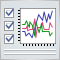如何分析图表中所选择信号的交易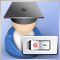包含图形用户界面 (GUI) 的 EA 交易: 创建面板 (第一部分)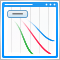改进面板：增加透明化、改变背景色以及继承于 CAppDialog/CWndClient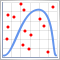蒙特卡洛方法在交易策略优化中的应用